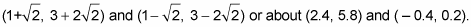##### Differential Equations For Dummies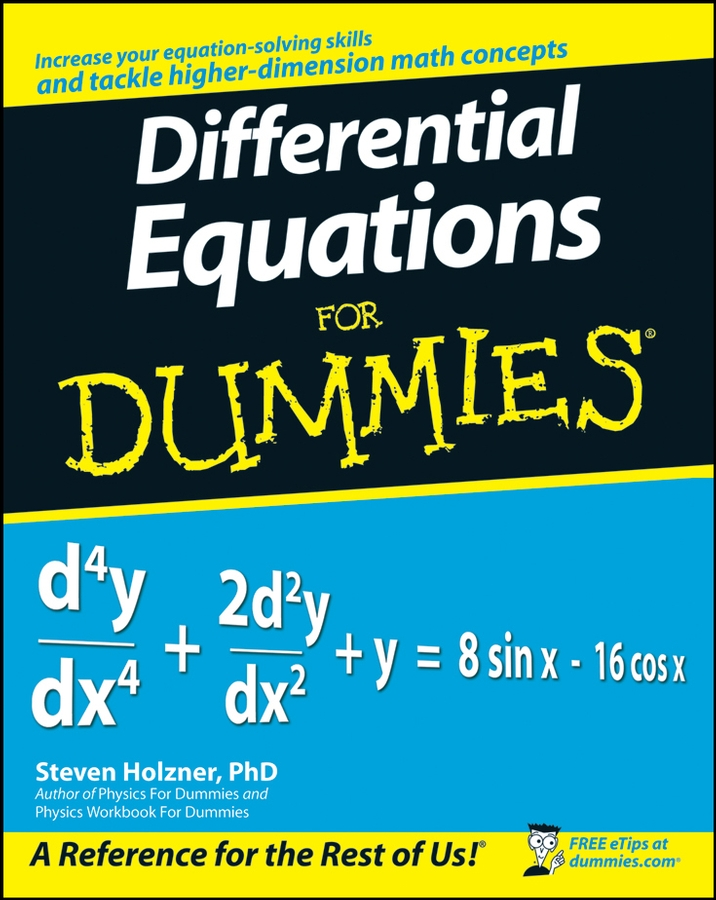Ever want to determine the location of a line through a given point that’s tangent to a given curve? Of course you have! Here’s how you do it.

Determine the points of tangency of the lines through the point (1, –1) that are tangent to the parabola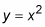If you graph the parabola and plot the point, you can see that there are two ways to draw a line that goes through (1, –1) and is tangent to the parabola: up to the right and up to the left (shown in the figure).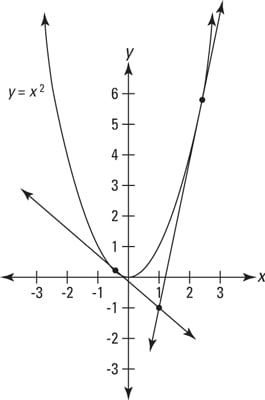The key to this problem is in the meaning of the derivative: The derivative of a function at a given point is the slope of the tangent line at that point. So, you just have to set the derivative of the parabola equal to the slope of the tangent line and solve:

1. Because the equation of the parabola is2. you can take a general point on the parabola, (x, y) and substitute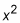3. for y.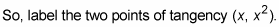4. Take the derivative of the parabola.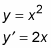5. Using the slope formula, set the slope of each tangent line from (1, –1) to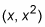6. equal to the derivative at7. which is 2x, and solve for x.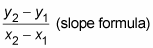By the way, the math you do in this step may make more sense to you if you think of it as applying to just one of the tangent lines — say the one going up to the right — but the math actually applies to both tangent lines simultaneously.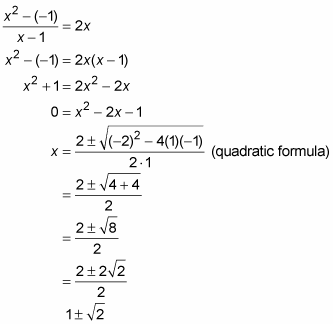So, the x-coordinates of the points of tangency are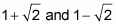8. Plug each of these x-coordinates into9. to obtain the y-coordinates.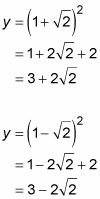Thus, the two points of tangency are